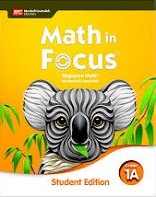1 Chapter 1 Numbers to 10 Lesson 1.1:  Counting to 10 Also consider: Lesson 1.1:  Counting to 10 Also consider: Lesson 1.2:  Comparing Numbers Also consider: Lesson 1.2:  Comparing Numbers Also consider: Lesson 1.3:  Number Patterns Lesson 1.3:  Number Patterns
 2 Chapter 2 Addition and Subtraction Within 10 Lesson 2.1:  Making Number Bonds Lesson 2.1:  Making Number Bonds Lesson 2.2:  Ways to Add Also consider: Lesson 2.2:  Ways to Add Also consider: Lesson 2.3:  Making Addition Stories Also consider: Lesson 2.3:  Making Addition Stories Also consider: Lesson 2.4:  Real-World Problems: Addition Lesson 2.4:  Real-World Problems: Addition Lesson 2.5:  Ways to Subtract Also consider: Lesson 2.5:  Ways to Subtract Also consider: Lesson 2.6:  Making Subtraction Stories Also consider: Lesson 2.6:  Making Subtraction Stories Also consider: Lesson 2.7:  Real-World Problems: Subtraction Lesson 2.7:  Real-World Problems: Subtraction Lesson 2.8:  Making Fact Families Also consider: Lesson 2.8:  Making Fact Families Also consider:
 3 Chapter 3 Shapes and Patterns Lesson 3.1:  Exploring Flat Shapes Also consider: Lesson 3.1:  Exploring Flat Shapes Also consider: Lesson 3.2:  Exploring Solid Shapes Lesson 3.2:  Exploring Solid Shapes Lesson 3.3:  Using Shapes to Make Pictures and Models Lesson 3.3:  Using Shapes to Make Pictures and Models Lesson 3.4:  Seeing Shapes Around Us Lesson 3.4:  Seeing Shapes Around Us Lesson 3.5:  Using Flat Shapes to Make Patterns Lesson 3.5:  Using Flat Shapes to Make Patterns Lesson 3.6:  Using Solid Shapes to Make Patterns Lesson 3.6:  Using Solid Shapes to Make Patterns
 4 Chapter 4 Numbers to 20 Lesson 4.1:  Counting to 20 Lesson 4.1:  Counting to 20 Lesson 4.2:  Place Value Lesson 4.2:  Place Value Lesson 4.3:  Comparing and Ordering Numbers Lesson 4.3:  Comparing and Ordering Numbers Lesson 4.4:  Number Patterns Lesson 4.4:  Number Patterns
 5 Chapter 5 Addition and Subtraction Within 20 Lesson 5.1:  Ways to Add Fluently Also consider: Lesson 5.1:  Ways to Add Fluently Also consider: Lesson 5.2:  Ways to Subtract Fluently Also consider: Lesson 5.2:  Ways to Subtract Fluently Also consider: Lesson 5.3:  Real-World Problems: Addition and Subtraction Also consider: Lesson 5.3:  Real-World Problems: Addition and Subtraction Also consider:
 6 Chapter 6 Numbers to 40 Lesson 6.1:  Counting to 40 Lesson 6.1:  Counting to 40 Lesson 6.2:  Place Value Lesson 6.2:  Place Value Lesson 6.3:  Comparing, Ordering, and Number Patterns Lesson 6.3:  Comparing, Ordering, and Number Patterns
 7 Chapter 7 Calendar and Time Lesson 7.1:  Using a Calendar Also consider: Lesson 7.1:  Using a Calendar Also consider: Lesson 7.2:  Telling Time to the Hour Lesson 7.2:  Telling Time to the Hour Lesson 7.3:  Telling Time to the Half Hour Also consider: Lesson 7.3:  Telling Time to the Half Hour Also consider:
 8 Chapter 8 Addition and Subtraction Within 40 Lesson 8.1:  Addition Without Regrouping Lesson 8.1:  Addition Without Regrouping Lesson 8.2:  Addition with Regrouping Lesson 8.2:  Addition with Regrouping Lesson 8.3:  Subtraction Without Regrouping Lesson 8.3:  Subtraction Without Regrouping Lesson 8.4:  Subtraction with Regrouping Lesson 8.4:  Subtraction with Regrouping Lesson 8.5:  Real-World Problems: Addition and Subtraction Lesson 8.5:  Real-World Problems: Addition and Subtraction Lesson 8.6:  Getting Ready for Multiplication Lesson 8.6:  Getting Ready for Multiplication
 9 Chapter 9 Length and Weight Lesson 9.1:  Comparing Lengths Lesson 9.1:  Comparing Lengths Lesson 9.2:  Comparing More Lengths Lesson 9.2:  Comparing More Lengths Lesson 9.3:  Using a Start Line Lesson 9.3:  Using a Start Line Lesson 9.4:  Measuring Length Lesson 9.4:  Measuring Length Lesson 9.5:  Measuring Length in Units Lesson 9.5:  Measuring Length in Units Lesson 9.6:  Comparing Weights Lesson 9.6:  Comparing Weights Lesson 9.7:  Measuring Weight Lesson 9.7:  Measuring Weight Lesson 9.8:  Measuring Weight in Units Lesson 9.8:  Measuring Weight in Units
 10 Chapter 10 Numbers to 120 Lesson 10.1:  Counting to 120 Also consider: Lesson 10.1:  Counting to 120 Also consider: Lesson 10.2:  Place Value Lesson 10.2:  Place Value Lesson 10.3:  Comparing, Ordering, and Number Patterns Also consider: Lesson 10.3:  Comparing, Ordering, and Number Patterns Also consider:
 11 Chapter 11 Addition and Subtraction Within 100 Lesson 11.1:  Addition Without Regrouping Also consider: Lesson 11.1:  Addition Without Regrouping Also consider: Lesson 11.2:  Addition with Regrouping Also consider: Lesson 11.2:  Addition with Regrouping Also consider: Lesson 11.3:  Subtraction Without Regrouping Lesson 11.3:  Subtraction Without Regrouping Lesson 11.4:  Subtraction with Regrouping Lesson 11.4:  Subtraction with Regrouping
 12 Chapter 12 Graphs Lesson 12.1:  Simple Picture Graphs Lesson 12.1:  Simple Picture Graphs Lesson 12.2:  Tally Charts and Picture Graphs Lesson 12.2:  Tally Charts and Picture Graphs
 13 Chapter 13 Money Lesson 13.1:  Penny, Nickel, and Dime Lesson 13.1:  Penny, Nickel, and Dime Lesson 13.2:  Quarter Also consider: Lesson 13.2:  Quarter Also consider: Lesson 13.3:  Counting Money Also consider: Lesson 13.3:  Counting Money Also consider: Lesson 13.4:  Adding and Subtracting Money Lesson 13.4:  Adding and Subtracting Money
This document includes the IXL® skill alignments to Houghton Mifflin Harcourt's Math in Focus curriculum. IXL provides skill alignments as a service to teachers, students, and parents. The skill alignments are provided by IXL and are not affiliated with, sponsored by, reviewed, approved or endorsed by Houghton Mifflin Harcourt or any other third party. IXL® and IXL Learning® are registered trademarks of IXL Learning, Inc. All other intellectual property rights (e.g., unregistered and registered trademarks and copyrights) are the property of their respective owners.